### Home > CC1 > Chapter 7 > Lesson 7.3.2 > Problem7-97

7-97.
1. Translate each of these situations into a variable expression such as those found in a magic number chart. Homework Help ✎

1. Pick a number and multiply it by 7.

2. Pick a number and divide it by 8.

3. Pick a number and reduce it by 10.

4. Pick a number, add 2, then multiply by 5.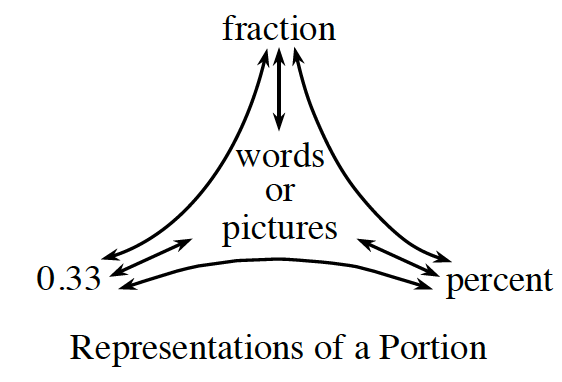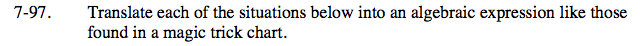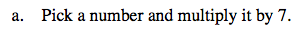Use x to represent the number you pick.

What would the expression look like if you multiplied your number by 7?

7x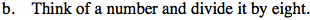See (a).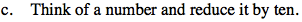What happens to a number when you reduce it by 10?

See part (a).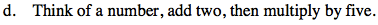See part (a).

5 ( x + 2 )